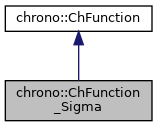chrono::ChFunction_Sigma Class Reference

## Description

Sigma function: y = polynomial smooth ramp

#include <ChFunction_Sigma.h>

Inheritance diagram for chrono::ChFunction_Sigma:[legend]
Collaboration diagram for chrono::ChFunction_Sigma:[legend]

## Public Member Functions

ChFunction_Sigma (double m_amp, double m_start, double m_end)

ChFunction_Sigma (const ChFunction_Sigma &other)

virtual ChFunction_SigmaClone () const override
"Virtual" copy constructor (covariant return type).

virtual FunctionType Get_Type () const override
Return the unique function type identifier.

virtual double Get_y (double x) const override
Return the y value of the function, at position x.

virtual double Get_y_dx (double x) const override
Return the dy/dx derivative of the function, at position x. More...

virtual double Get_y_dxdx (double x) const override
Return the ddy/dxdx double derivative of the function, at position x. More...

void Set_start (double m_start)

void Set_end (double m_end)

void Set_amp (double m_amp)

double Get_start () const

double Get_end () const

double Get_amp () const

virtual double Get_Ca_pos () const override
Computes the positive acceleration coefficient (inherited classes should customize this).

virtual double Get_Ca_neg () const override
Compute the positive acceleration coefficient (inherited classes should customize this).

virtual double Get_Cv () const override
Compute the speed coefficient (inherited classes must customize this).

virtual void Estimate_x_range (double &xmin, double &xmax) const override
Return an estimate of the range of the function argument. More...

virtual void ArchiveOUT (ChArchiveOut &marchive) override
Method to allow serialization of transient data to archives.

virtual void ArchiveIN (ChArchiveIn &marchive) override
Method to allow de-serialization of transient data from archives.Public Member Functions inherited from chrono::ChFunction
ChFunction (const ChFunction &other)

virtual double Get_weight (double x) const
Return the weight of the function (useful for applications where you need to mix different weighted ChFunctions)

virtual void Estimate_y_range (double xmin, double xmax, double &ymin, double &ymax, int derivate) const
Return an estimate of the range of the function value. More...

virtual double Get_y_dN (double x, int derivate) const
Return the function derivative of specified order at the given point. More...

virtual void Update (const double x)
Update could be implemented by children classes, ex. to launch callbacks.

virtual double Compute_max (double xmin, double xmax, double sampling_step, int derivate) const
Compute the maximum of y(x) in a range xmin-xmax, using a sampling method.

virtual double Compute_min (double xmin, double xmax, double sampling_step, int derivate) const
Compute the minimum of y(x) in a range xmin-xmax, using a sampling method.

virtual double Compute_mean (double xmin, double xmax, double sampling_step, int derivate) const
Compute the mean value of y(x) in a range xmin-xmax, using a sampling method.

virtual double Compute_sqrmean (double xmin, double xmax, double sampling_step, int derivate) const
Compute the square mean val. of y(x) in a range xmin-xmax, using sampling.

virtual double Compute_int (double xmin, double xmax, double sampling_step, int derivate) const
Compute the integral of y(x) in a range xmin-xmax, using a sampling method.

virtual int HandleNumber () const
Return the number of handles of the function.

virtual bool HandleAccess (int handle_id, double mx, double my, bool set_mode)
Get the x and y position of handle, given identifier. More...

virtual int FilePostscriptPlot (ChFile_ps *m_file, int plotY, int plotDY, int plotDDY)
Plot function in graph space of the ChFile_ps postscript file where zoom factor, centering, color, thickness etc. More...

virtual int FileAsciiPairsSave (ChStreamOutAscii &m_file, double xmin=0, double xmax=1, int msamples=200)
Save function as X-Y pairs separated by space, with CR at each pair, into an ASCII file. More...

## Additional Inherited MembersPublic Types inherited from chrono::ChFunction
enum  FunctionType {
FUNCT_CUSTOM, FUNCT_CONST, FUNCT_CONSTACC, FUNCT_DERIVE,
FUNCT_FILLET3, FUNCT_INTEGRATE, FUNCT_MATLAB, FUNCT_MIRROR,
FUNCT_MOCAP, FUNCT_NOISE, FUNCT_OPERATION, FUNCT_OSCILLOSCOPE,
FUNCT_POLY, FUNCT_POLY345, FUNCT_RAMP, FUNCT_RECORDER,
FUNCT_REPEAT, FUNCT_SEQUENCE, FUNCT_SIGMA, FUNCT_SINE,
FUNCT_LAMBDA
}
Enumeration of function types.

## ◆ Estimate_x_range()

 void chrono::ChFunction_Sigma::Estimate_x_range ( double & xmin, double & xmax ) const
overridevirtual

Return an estimate of the range of the function argument.

(Can be used for automatic zooming in a GUI)

Reimplemented from chrono::ChFunction.

## ◆ Get_y_dx()

 double chrono::ChFunction_Sigma::Get_y_dx ( double x ) const
overridevirtual

Return the dy/dx derivative of the function, at position x.

Note that inherited classes may also avoid overriding this method, because this base method already provide a general-purpose numerical differentiation to get dy/dx only from the Get_y() function. (however, if the analytical derivative is known, it may better to implement a custom method).

Reimplemented from chrono::ChFunction.

## ◆ Get_y_dxdx()

 double chrono::ChFunction_Sigma::Get_y_dxdx ( double x ) const
overridevirtual

Return the ddy/dxdx double derivative of the function, at position x.

Note that inherited classes may also avoid overriding this method, because this base method already provide a general-purpose numerical differentiation to get ddy/dxdx only from the Get_y() function. (however, if the analytical derivative is known, it may be better to implement a custom method).

Reimplemented from chrono::ChFunction.

The documentation for this class was generated from the following files:
• /builds/uwsbel/chrono/src/chrono/motion_functions/ChFunction_Sigma.h
• /builds/uwsbel/chrono/src/chrono/motion_functions/ChFunction_Sigma.cpp